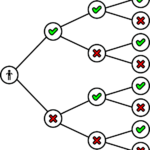# Understanding Algorithm Analysis and Design

Algorithm Analysis and Design refers to the calculation and prediction of resources that an algorithm requires. Moreover, this includes time complexity and space complexity.

We require analysis to know so that we easily find the most efficient algorithm. Certainly, efficiency of an algorithm is a measure of amount of resources that it consumes to solve a problem of size n.

There are two approaches:

Firstly, Empirical approach deals with running an algorithm and measure time. Secondly, theoretical approach deals with mathematical computing.

### Characteristics of Algorithm Analysis

Algorithm is a collection of instructions to produce an output in a specific time. Let us see some of its charateristics:

• Finiteness: Firstly, an algorithm should have finite number of steps.
• Definiteness: Secondly, steps must be precisely defined.
• Input and Output : Thirdly, an algorithm should have 0 or more inputs and at least one output.
• Effectiveness: It must be efficiently written.

### Problem development steps

• Understanding problem definition
• Specify an algorithm
• Check the correctness of algorithm
• Analysis
• Implementation of algorithm
• Program testing
• Documentation

### Significance of Algorithm Analysis

1. Firstly, to check the correctness and efficiency before implementing it.
2. Secondly, to focus on techniques for solving complex problems.
3. Thirdly, to understand relationship between different computational problems.

### Asymptomatic Notations

Asymptomatic notations are the notations like Big O, Theta and Omega which are used to analyze an algorithm’s running time and its growth rate.

Lower Bound: It deals with minimum time that an algorithm requires. Upper Bound: It deals with maximum time that an algorithm requires.

Big O Notation is asymptomatically Upper Bound (U(n)) for f(n). Moreover, Omega Notation is asymptomatically (L(n)) for f(n).

### Amortized Analysis

This analysis takes place where an occasional operation is slow but almost all other operations are fast. We analyze sequence of operations. And guarantee a worst case average time lower than a worst case time of an expensive operation.

It shows average case of an operation is small even though a single operation is expensive.

#### Techniques:

• Aggregate Method
• Accounting
• Potential Method

In aggregate method, sequence of n operation with assuming worst time T(n). Cost per operation is T(n)/n.

In accounting, assume each type of operation with different cost.

In potential method, store credit in potential form and as a whole.

### Summary

In conclusion, we have learnt that algorithm analysis and design refers to the calculation and prediction of resources that an algorithm requires. We require analysis to know so that we easily find the most efficient algorithm. Certainly, efficiency of an algorithm is a measure of amount of resources that it consumes to solve a problem of size n.

We have learnt about is characteristics and its significance. Also we know the asymptomatic notations like Big O, Theta and Omega.#### Drishti Patel

View all posts
0 0 vote
Article Rating
Subscribe
Notify of